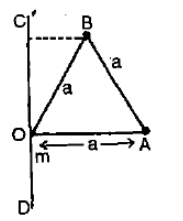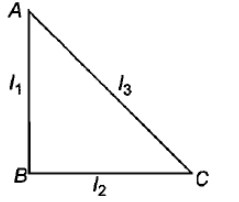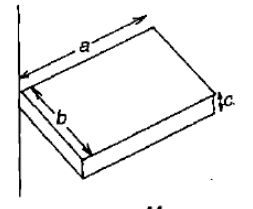Three-point masses 'm' each, are placed at the vertices of an equilateral triangle of side a. Moment of inertia of the system through a mass m at O and lying in the plane of COA & perpendicular to OA is1. $2m{a}^{2}$

2. $\frac{2}{3}m{a}^{2}$

3. $\frac{5}{4}m{a}^{2}$

4. $\frac{7}{4}m{a}^{2}$

Concept Questions :-

Moment of inertia
High Yielding Test Series + Question Bank - NEET 2020

Difficulty Level:

ABC is a right angled triangular plate of uniform thickness. The sides are such that AB > BC as shown in figure.  and ${\mathrm{I}}_{3}$ are moments of inertia about AB, BC and AC respectively. Then, which of the following relation is correct?Concept Questions :-

Moment of inertia
High Yielding Test Series + Question Bank - NEET 2020

Difficulty Level:

One solid sphere A and another hollow sphere B are of same mass and same outer radii. Their moment of inertia about their diameters are respectively IA and IB such that

1. ${\mathrm{I}}_{\mathrm{A}}={\mathrm{I}}_{\mathrm{B}}$

2. ${\mathrm{I}}_{\mathrm{A}}>{\mathrm{I}}_{\mathrm{B}}$

3. ${\mathrm{I}}_{\mathrm{A}}<{\mathrm{I}}_{\mathrm{B}}$

4. $\frac{{\mathrm{I}}_{\mathrm{A}}}{{\mathrm{I}}_{\mathrm{B}}}=\frac{{d}_{A}}{{d}_{B}}$

Concept Questions :-

Moment of inertia
High Yielding Test Series + Question Bank - NEET 2020

Difficulty Level:

For the same total mass, which of the following will have the largest moment of inertia about an axis passing through the centre of gravity and perpendicular to the plane of the body?

1. A disc of radius a

2. a ring of radius a

3. a square lamina of side 2 a

4. four rods forming square of side 2 a.

Concept Questions :-

Moment of inertia
High Yielding Test Series + Question Bank - NEET 2020

Difficulty Level:

The radius of gyration of a uniform rod of length L about an axis passing through its centre of mass is

1. $\frac{L}{2\sqrt{3}}$

2. $\frac{{L}^{2}}{12}$

3. $\frac{L}{\sqrt{3}}$

4. $\frac{L}{\sqrt{2}}$

Concept Questions :-

Moment of inertia
High Yielding Test Series + Question Bank - NEET 2020

Difficulty Level:

Two uniform, thin identical rods each of mass M and length l are joined together to form a cross. What will be the moment of inertial of the cross about an axis passing through the point at which the two rods are joined and perpendicular to the plane of the cross

1. $\frac{M{l}^{2}}{12}$

2. $\frac{M{l}^{2}}{6}$

3. $\frac{M{l}^{2}}{4}$

4. $\frac{M{l}^{2}}{3}$

Concept Questions :-

Moment of inertia
High Yielding Test Series + Question Bank - NEET 2020

Difficulty Level:

The moment of inertia of a rod about an axis through its centre and  perpendicular to it is $\frac{1}{12}M{L}^{2}$ (where M is the mass and L is the length of the rod). The rod is bent in the middle so that the two halves make an angle of 60$°$. The moment of inertia of the bent rod about the same axis would be

(1) $\frac{1}{48}M{L}^{2}$

(2) $\frac{1}{12}M{L}^{2}$

(3) $\frac{1}{24}M{L}^{2}$

(4) $\frac{M{L}^{2}}{8\sqrt{3}}$

Concept Questions :-

Moment of inertia
High Yielding Test Series + Question Bank - NEET 2020

Difficulty Level:

One circular ring and one circular disc both having the same mass and radius. The ratio of their moments of inertia about the axes passing through their centres and perpendicular to planes will be

1. 1:1

2. 2:1

3. 1:2

4. 4:1

Concept Questions :-

Moment of inertia
High Yielding Test Series + Question Bank - NEET 2020

Difficulty Level:

The figure shows a uniform solid block of mass M and edge lengths a, b and c. Its M.O.I. about an axis through one edge and perpendicular (as shown) to the large face of the block is1. $\frac{M}{3}\left({a}^{2}+{b}^{2}\right)$

2. $\frac{M}{4}\left({a}^{2}+{b}^{2}\right)$

3. $\frac{7M}{12}\left({a}^{2}+{b}^{2}\right)$

4. $\frac{M}{12}\left({a}^{2}+{b}^{2}\right)$

Concept Questions :-

Moment of inertia
High Yielding Test Series + Question Bank - NEET 2020

Difficulty Level:

The moment of inertia of a cube of mass m and side a about one of its edges is equal to

(1)                 (2)

(3)                   (4)

Concept Questions :-

Moment of inertia